### General Physics, High School and College Freshman level### A Tutorial on The Basics of Reflection: Notes, Figures and Problems with Solutions

Target Audience: High School Students, College Freshmen and Sophomores, students preparing for the International Baccalaureate (IB), AP Physics B, AP Physics C, A Level, Singapore/GCE A-Level;

## Reflection is basically the phenomenon due to which a light ray reverses its direction on striking a different optical medium. It occurs in different areas of nature, namely light, waves, signal scattering, SONAR reflection, so on and so forth. We will come across reflection in another module of our learning stride -- Wave Optics.

### Reflection at plane surfaces

(i) Reflection of Light

When light rays strike the boundary of two media such as air and glass, a part of light is turned back into the same medium. This is called Reflection of Light. The wavelength and the velocity of the light wave remains the same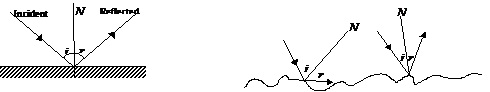Laws of reflection are obeyed at every reflecting surface, i.e.

(ii) Laws of Reflection

(a)       The incident ray (AB), the reflected ray (BC) and normal (BN) to the surface (SS') of reflection at the point of incidence (B) lie in the same plane. This plane is called the plane of incidence (or plane of reflection).

(b)       The angle of incidence (the angle between normal and between the reflected ray and the normal are equal, i.e. ∠i = ∠r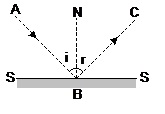((iii) Types of reflection

(a)    Regular Reflection    (b)    Diffused Reflection or Scattering or Diffusion

(a)    Regular Reflection: When the reflection takes place from a perfect plane surface it is called Regular Reflection.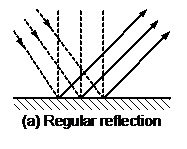(b)    Diffused Reflection or Scattering or Diffusion: When the surface is rough light is reflected from the surface from bits of its plane surfaces in irregular directions. This is called diffusion. This process enables us to see an object from any position.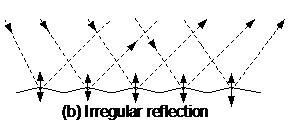### Characteristics of Reflection by a Plane Mirror

Absorb each of the following concepts slowly and grasp them with the help of a diagram for each. These are the building blocks of the entire reflection concept and will be very useful in solving problems.

(i)    Distance of object from mirror = Distance of image from the mirror.

(ii)    The line joining the object point with its image is normal to the reflecting surface.

(iii)    The image is laterally inverted (left-right inversion).

(iv)    The size of the image is the same as that of the object.

(v)    For a real object the image is virtual and for a virtual object the image is real.

(vi)    The minimum size of a plane mirror, required to see the full self image, is half the size of that person.

(vii)    For a light ray incident at an angle 'i' after reflection angle of deviation d = π -2i

(viii)    If i = 0 then r = 0, this implies that a ray of light incident normally on a mirror retraces its path.

(ix)    The eye always observes an object in the direction in which the rays enter the eye

(x)    The laws of reflection holds good for all kinds of reflection.

(xi)    Image of an object is the point at which rays after reflection (or reflection) actually converge or appear to diverge from that point.

(xii)    If the direction of the incident ray is kept constant and the mirror is rotated through an angle θ about an axis in the plane mirror then the reflected ray rotates through an angle 2 θ.

(xiii)    If an object moves towards (or away from) a plane mirror at a speed v, the image will also approach (or recede) at the same speed.

Further the relative speed of image to the object will be v – (–v) = 2v.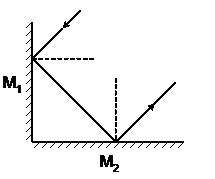(xiv) If two plane mirror are inclined to each other at 90o, the emergent ray is always antiparallel to incident ray, if reflected from each mirror, irrespective of angle of incident.

(xv)When two plane mirror, inclined to each other at an angle θ, the number of images formed can be determined as follows:

(a)    If  (360/θ) is an even integer, say ‘p’, then number of image formed say q = p – 1, for all position.

(b)    If (360/θ)  is an odd integer say ‘q’ then number of image formed say p = q, if the object is not on the bisector of the angle between mirrors. Also, p = q – 1, if the object is on the bisector.

(c)    If  (360/θ) is a fraction, then the number of image formed will be equal to its integral part.

(xvi)    The images are laterally inverted.

(xvii)    The linear magnification is unity.

m=(-v/u)

=> |m|=1.

(As the object distance is equal to image distance, u=v).

### REFLECTION-AT SPHERICAL MIRRORS

A spherical mirror is a reflecting surface which forms a part of a sphere (as shown in following a and b diagram). When the reflection takes place from the inner surface and outer surface is polished or silvered the mirror is known as concave mirror. Vice- versa, it is convex.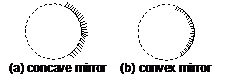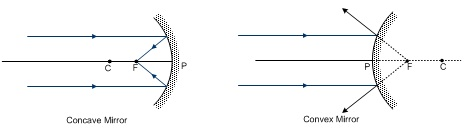Ray diagram for concave and convex mirror

### (FOR SMALL APERTURE)

(i)    Pole (P) is generally taken as the midpoint of reflecting surface.

(ii)    Centre of curvature (C) is the centre of the sphere of which the mirror is a part.

(iii)    Radius of curvature is the radius of the sphere of which the mirror is a part. Distance between P and C.

(iv)    Principal Axis is the straight line connecting pole P and centre of curvature C.

(v)    Principal focus (F) is the point of intersection of all the reflected rays which strike the mirror (with small aperture) parallel to the principal axis. In concave mirror it is real and in the convex mirror it is virtual.

(vi)    Focal length (f) is the distance from pole to focus.

(vii)    Aperture is the diameter of the mirror.

(viii)    If the incident ray is parallel to the principal axis, the reflected ray passes through the focus. (Fig (a))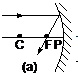(ix)    If the incident ray passes through the focus, then the reflected ray is parallel to the principal axis (Fig.(b))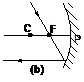(x)    Incident ray passing through centre of curvature will be reflected back through the centre of curvature. (Fig.(c))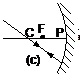(xi)     F = R/2,  where f = focal length R = Radius of curvature

(xii)    Sign convention and magnification. We follow Cartesian coordinate system convention according to which:

(a)    The pole of the mirror is the origin

(b)    The direction of the incident rays is considered as positive x-axis.

(c)    Vertically up is positive y-axis

Note: Radius of Curvature and Focal Length of:

(a)    Concave mirror is positive

(b)    Convex mirror is positive

(xiii)   (a)    Linear Magnification or lateral magnification or transverse magnification

= M = h2 / h1 = - v/u

where h2 = y  coordinate of image;  h1 = y coordinate of the object

(both perpendicular to the principle axis of mirror)

(b)    Longitudinal Magnification  (for any size of the object)  (for short object)

### Tracing for spherical mirror

(1)    A ray going through centre of curvature is reflected back along the same direction.

(2)    A ray parallel to principal axis is reflected through the focus, and vice-versa. Also, mutually parallel rays after reflection intersect on the focal plane.

(3)    A ray going to the pole and the reflected ray from it make equal angles with the principal axis.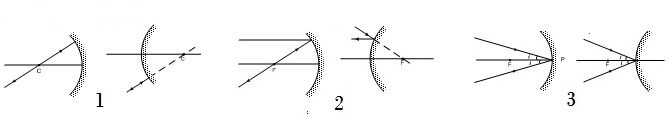The velocities of the object and image can be related using the relation:

1/v + 1/u = 1/f

where:

v .... Image Distance

u .... Object Distance

f .... Focal Length of the mirror.

Use the conventions for the sign of focal length for a convex/ concave mirror appropriately.

Simple Problems on Reflection

A list of Solved Examples.

1. A person runs with a speed u towards a bicycle moving away from him at the speed v. The person approaches his image in the plane mirror fixed at the rear of the bicycle with a speed of

1. U-v

2. U-2v

3. 2u-v

4. 2(u-v)

Solution: Consider the motion of the person keeping the bicycle stationary. Hence, the person runs towards him at the speed of (u-v).Now, for a plane mirror, the image moves with the same speed as the  object, but in the opposite direction, hence the image approaches the object as well with velocity –(u-v). Hence, the object approaches the image with velocity (u-v)-(u-v) =2(u-v).

1. Is the formula 1/v + 1/u = 1/f valid for a simple mirror also?

Solution: This is a general formula for all spherical mirrors. For flat mirrors

R=∞ , as it can be assumed to be a part of a sphere with infinite radius, then this equation can be used.It yields v = -u.

1. A ray of light on a plane mirror along a vector i+j+k. The normal on incident point is along i+j . Find a unit vector along the reflected ray?

r = R/ |R| = (-i -j -k)/√3

1. The change in phase of the reflected ray, when the light wave suffers reflection at air-glass interface:

1. Pi

2. 2pi

3. 0

4. Pi/2

Solution: When  a wave suffers refraction from a rarer to a denser medium, then the phase changes by ‘pi’ as the direction of the wave changes to the opposite direction. Hence, the answer is pi.

1. A boy of length 10 m, to see his own complete image, requires a mirror of length at least equal to :

1. 10 m

2. 5 m

3. 3.33 m

4. 2 m

Solution: A person requires the mirror to be at least half his/her height for the entire body to be visible. Hence, the answer would be 10/2 m, which is 5m.

6. A plane mirror is in front of you in which you can see your image. It is      approaching towards you at a speed of 10 cm/s, then at what speed will your image approach you ?

a) 10 cm/s

b) 5 cm/s

c) 20 cm/s

d) 15 cm/s

Solution: For a plane mirror, the object velocity is equal and opposite to the image velocity. Hence, if the mirror is moving with a velocity v, then the relative velocity between the two is 2v, which in this case is 2*10=20 cm/s.

1. A plane mirror is placed along positive x-axis facing along positive y-axis. The equation of a linear object is x =y. The equation of its image is:

1. X = y

2. X+y =0

3. 2x+y=0

4. None of the above

Solution: The mirror reflection of the line x=y about the x-axis will be the line y + x = 0. This can be effectively visualized by the graph of y=x and y=-x.

8. A plane mirror :

(i) can form real image of a real object

(ii) neither converges nor diverges the rays

(iii) cannot form real image of a real object Choose the correct option or options :

(a) (i) is correct

(b) (i) and (ii) are correct

(c) (ii) and (iii) are correct.

(d) none of the above

Solution: The position of image is the point of divergence or convergence of reflected rays. If light from a real object incident on a plane mirror, the rays incident will be diverging. As power of the plane mirror is zero, hence, it neither converges nor diverges the rays.

9 .Convergence of concave mirror can be decreased by dipping in :

1. Water

2. Oil

3. Both a) and b)

4. None .

Solution: The convergence capability of a lens can be gauged from the difference in refractive indices of a lens. Hence, either when dipped in water or oil, the lens’ convergence can be decreased effectively.

10. The focal length of convex mirror is 20 cm, its radius of curvature           will be :

a) 30 cm

b) 45 cm

c) 40 cm

d) 80 cm

Solution: The focus is located at R/2 distance from the spherical mirror. This distance is called focal length. Hence, f = R/2. Thus, R=2f.

11. What will be the deviation produced in the ray, if a ray of light incidents on a plane mirror at an angle of 30° ?

a) 30°

b) 60°

c) 90°

d) 120°

Solution: When a light ray is incident on a plane mirror at angle β, the deviation produced by the light ray is pi-2β. Hence, in this case, 180°-60°=120°.

12 .Image formed by convex mirror is :

1. Virtual

2. Enlargened

3. Real

4. Diminished

Solution: The image formed by a convex mirror is always virtual, never real. Also, the images are always diminished. Hence, both the options are correct.

## MCQ Quiz on Reflection

#### MCQ Quiz: Reflection

This compilation of notes has been prepared by Anirud Thyagarajan of IIT Kharagpur